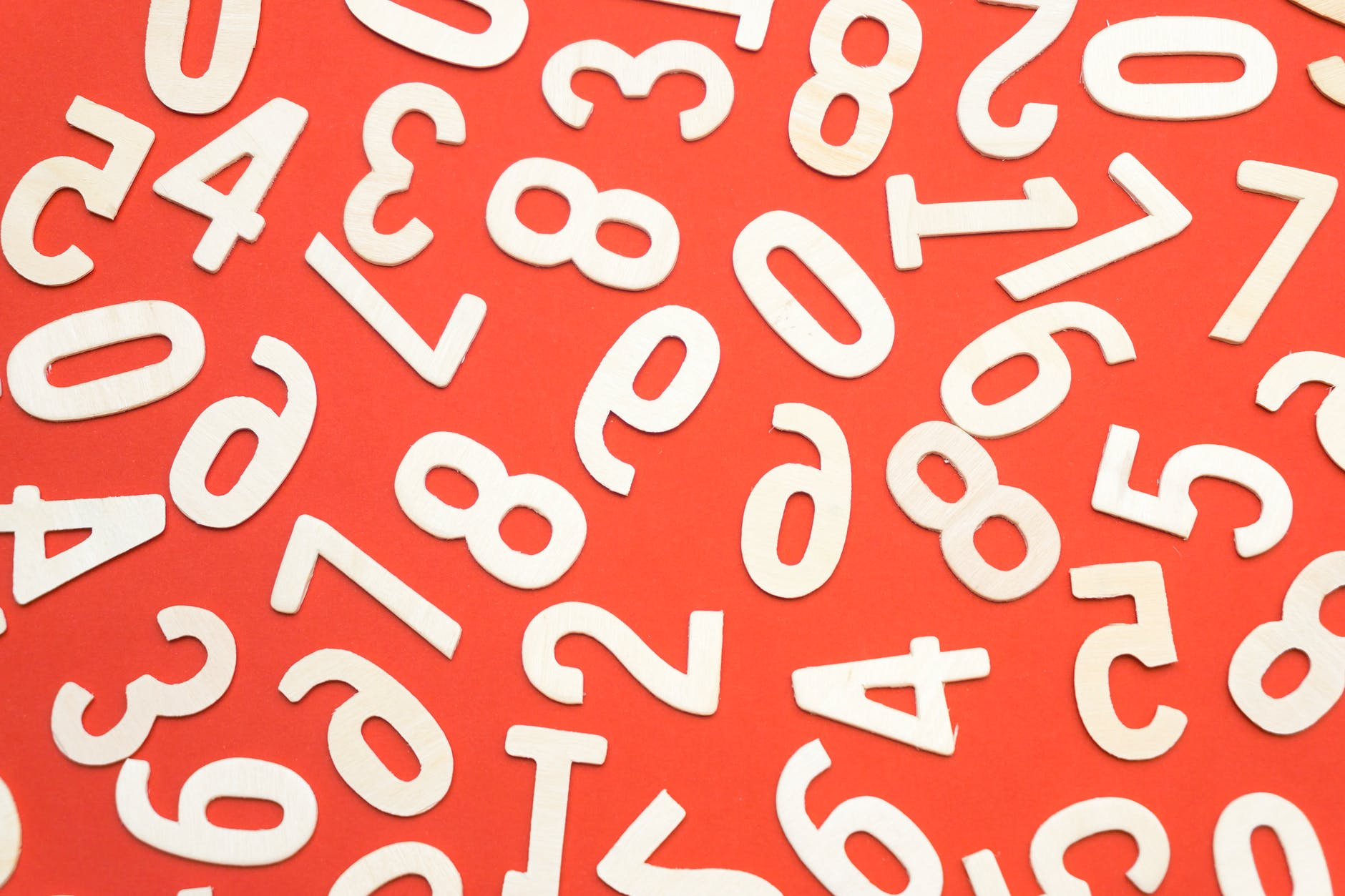# More Extra Math Questions# Extra Math Questions# Distance# Total Cost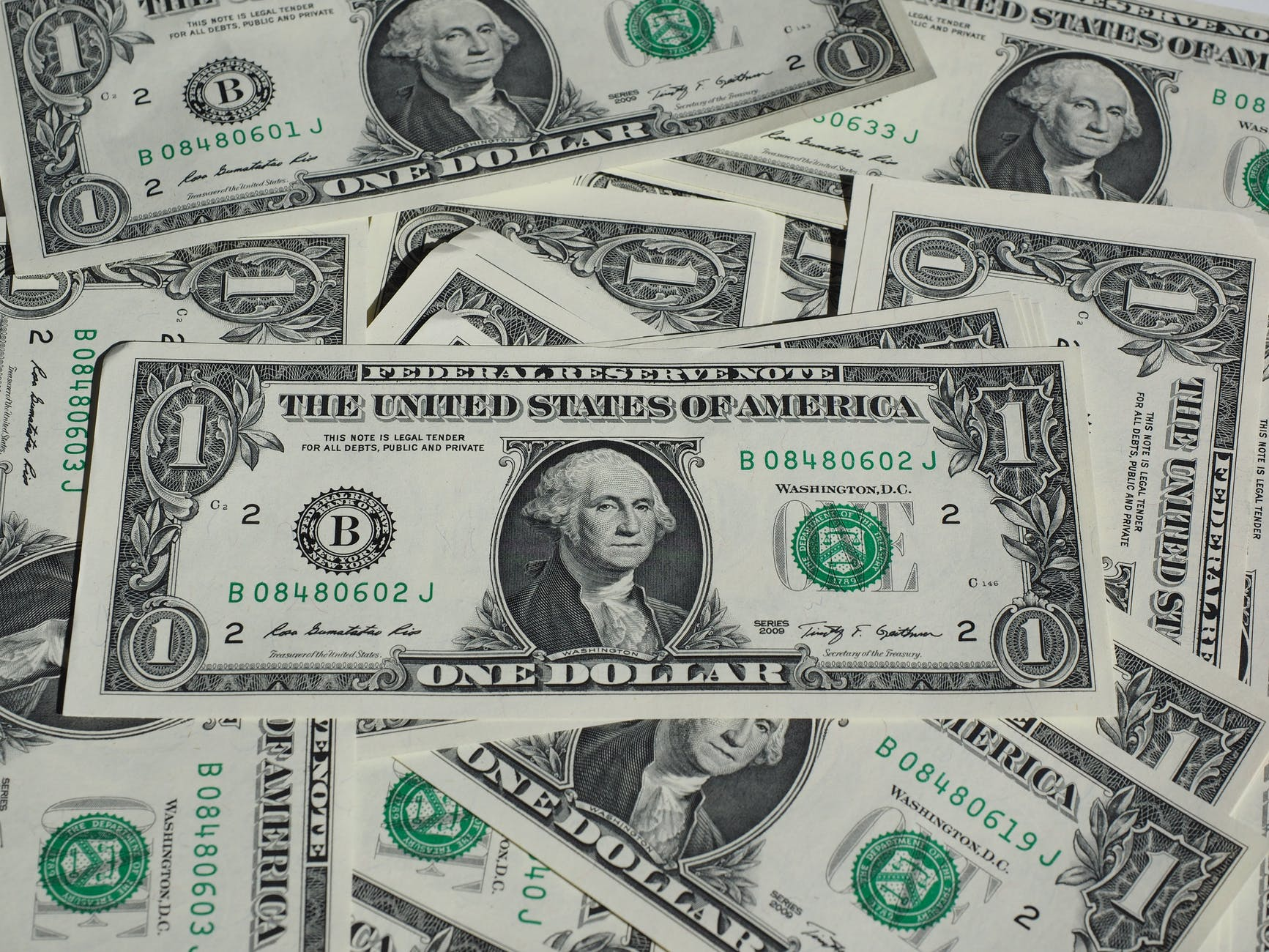# Point-Slope

Point-Slope Form of the Equation of a Line
point-slope form of the equation of a line
y – y1 = m(x – x1)

# Probability# Slope-Intercept# Slope

Slope refers to the Steepness of a Line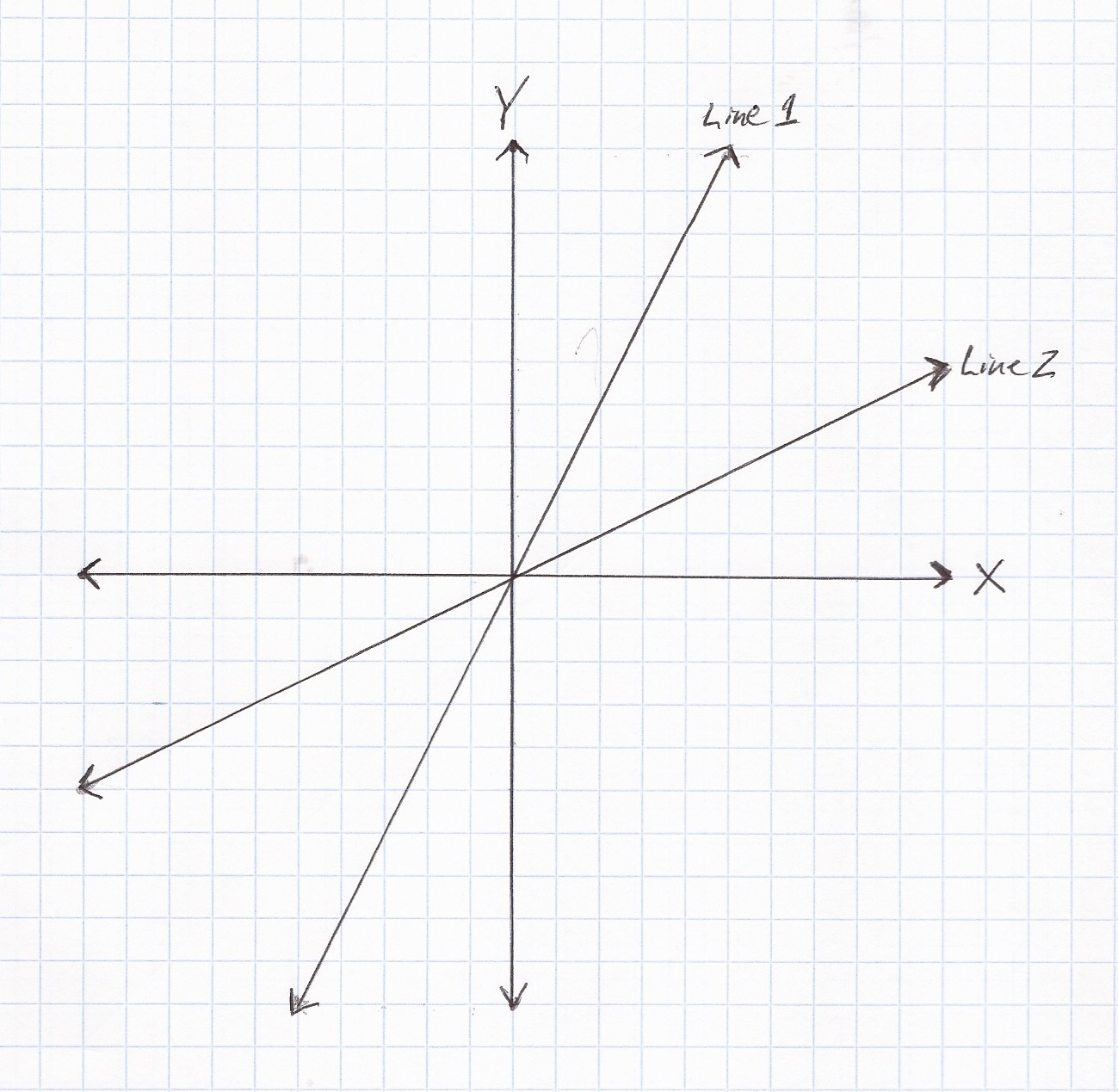# Line# Pythagorean Theorem

### a²  +  b²  =  c²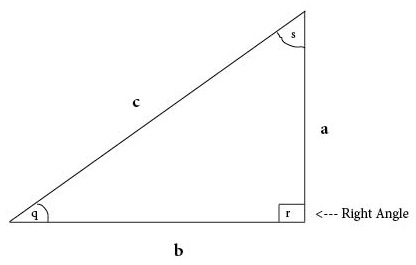# Simple Interest# Parallelogram

Parallelogram = Flat Four-Sided Shape in which Opposite Sides are Parallel

# Trapezoid

Trapezoid = Flat Four-Sided Shape with Two Parallel Sides

# Cylinder

Cylinder = Three-Dimensional Shape with Circular Top and Base# Right Rectangular Prism

Right Rectangular Prism = Three-Dimensional Shape in which Opposite Faces are Equal (with all right angles)# Sphere

Sphere = 3-Dimensional Round Shape with All Surface Points Equidistant from Center# Mode

Mode = Most Frequent Number

# Mean

Mean =$\bf\displaystyle\frac{Sum}{Count}$

# Triangle

Triangle = Flat Shape with Three Sides# Rectangle

Rectangle = Flat Shape with Four Sides (with four right angles)

# Cube Root

Cube Root = ³Number³ = ³Number × Itself × Itself

# Cubing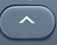3

Number Cubed = Number × Itself × Itself = Number³

# Cube

Cube = Three-Dimensional Shape with Six Square Faces (with all right angles)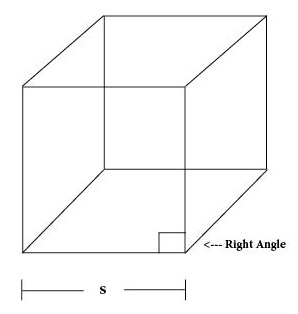# Square

Square = Flat Shape with Four Equal Sides (with four right angles)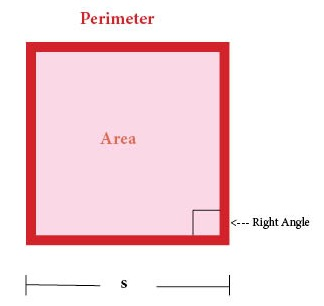# Square Root

Square Root =  Number² = √ Number × Itself

# SquaringNumber Squared = Number × Itself = Number²